# Three Dimensional (3D) Shapes

### LESSON – 3D SHAPES

###### TOPIC
• Introduction
• Meaning and Properties of Three Dimensional Shape
• Lesson Evaluation

###### INTRODUCTION – 2D AND 3D SHAPES

Three dimensional shapes are objects or shapes that three dimensions such as length, width, and height.

Three dimensional shapes are known as 3D shapes.

While two dimensional shapes have just two dimensions such as length and width.

Two dimensional shapes are also known as 2D shapes.

The properties of a three dimensional shapes are,

1. Faces

2. Edges

3. Vertices

###### TYPES OF THREE DIMENSIONAL SHAPES

The following shapes are the basic three dimensional shapes we see around us,

1. Cube

2. Cuboid (Rectangular prism)

3. Triangular prism

4. Sphere

5. Cone

6. Cylinder

7. Pyramid

###### CUBE

A cube is a solid three dimensional shape which has 6 square faces.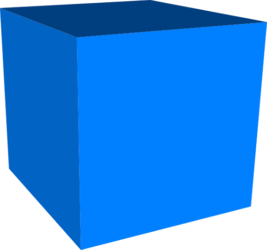Examples of cube are ice cube, cube square, dice, etc.

The cube has the following properties,

• 8 vertices (corners)
• 12 edges
• 6 faces

###### CUBOID

A cuboid is a solid three dimensional shape which has 6 rectangular faces or 4 rectangular faces and 2 square faces.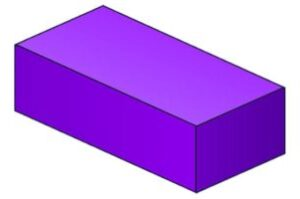Six rectangular faces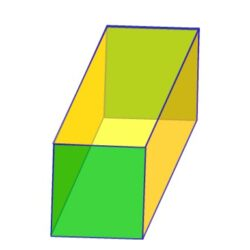Four rectangular faces with two square faces

Examples of cuboid are the lunch box, bricks, books, carton, cupboard, fridge, etc.

It is also called a rectangular prism.

The faces of the cuboid are a rectangle in shape.

The cuboid has the following properties,

• 8 vertices (corners)
• 12 edges
• 6 f aces

###### TRIANGULAR PRISM

A triangular prism is a solid three dimensional shape which has 5 faces (2 triangles and 3 rectangles).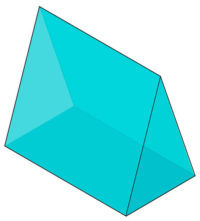Examples of triangular prism are triangular roofs, camping tents, and chocolate candy bars.

The triangular prism has the following properties,

• 6 vertices
• 9 edges
• 5 faces

###### SPHERE

A sphere is a three dimensional round shaped object with just one face.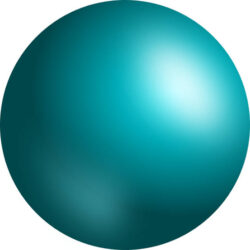Examples of sphere ball, moon, sun, eyeball, orange, etc.

The sphere has the following properties,

• No vertex
• No edges
• 1 curved face

###### CONE

A cone is a three dimensional object or solid with a circular base and has a single vertex.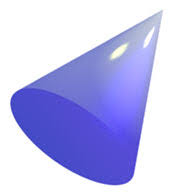Examples of cone are hat, Ice cream cone, funnel, megaphone, christmas tree, traffic cone, etc.

The cone has the following properties,

• 1 vertex
• 1 edge
• 1 flat face – circle
• 1 curved face

###### CYLINDER

A cylinder is defined as a three dimensional shapes with 2 circular bases connected by a curved surface.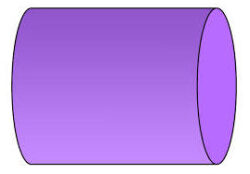Examples of cylinder pipes, cans, tanks, battery, gas cylinder, fire extinguisher, etc.

The cylinder has the following properties,

• No vertex
• 2 edges
• 2 flat faces – circles
• 1 curved face

###### PYRAMID

A pyramid is also a three dimensional shape with a square base and four triangular faces.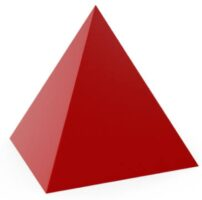Examples of pyramid are roof of a building, Egyptian pyramid, groundnut pyramid, etc.

There are different types of pyramid depending on their based.

We have the triangular, square, quadrilateral base but the most commonly used is the square pyramid.

The pyramid has the following properties,

• 5 vertices
• 8 edges
• 5 faces

###### LESSON EVALUATION

Answer 5 questions including number 6.

1. explain the meaning of 3 dimensional shapes.

2. differentiate between 2D and 3D shapes.

3. identify and state the properties of 3 dimensional shapes.

4. state the uses of three dimensional shapes.

5. apply 3 dimensional shapes in real life situations.

6. state the properties of the following shapes,

• Cube
• Cuboid
• Triangular prism
• Cone
• Sphere
• Pyramid
• Cylinder